# State-Space Averaging Method － From Another Viewpoint

2017.10.26

・Equations derived using state-space averaging are used to derive the dynamic states Δvout/ΔD and ΔiL/ΔD.

・With an understanding of the basic principles for equation derivation, it is possible to similarly derive Δvout/ΔD、ΔiL/ΔD starting from a different viewpoint.

In the previous section, we used dynamic analysis to derive equations enabling the derivation of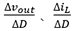, for the purpose of state-space averaging. This time, we will use an approach based on a different viewpoint to derive the equations derived previously. This depends on an understanding of the basic principles used in equation derivations thus far. The equations and procedures of the previous section are necessary, and are used here again.

The following is the dynamic analysis described the last time. These are equations for the coil current and capacitor charge derived previously. Please review the analysis once again.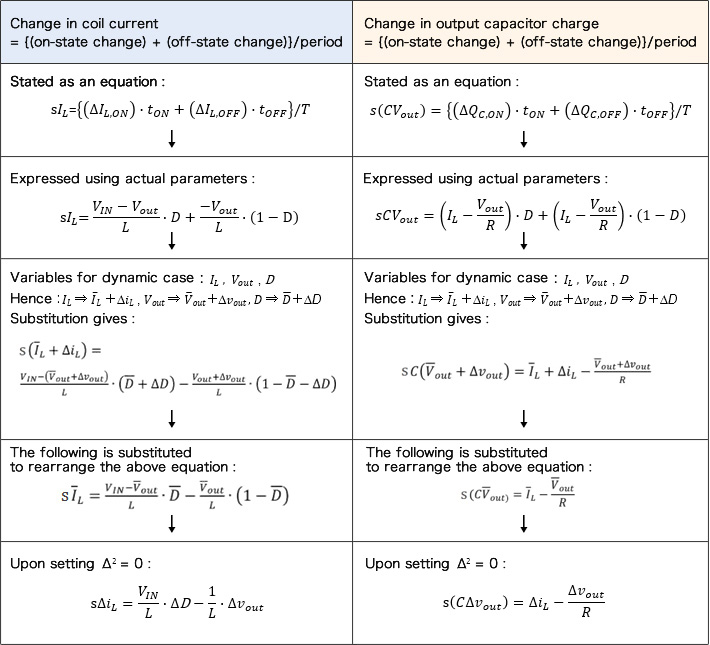The final equations are written similarly to the last time, and as was explained the last time, if these equations are simultaneous, thencan be determined.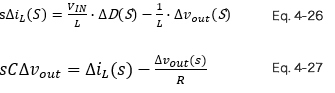From here we embark on this new approach. The following equations 4-28 and 4-29 represent the impedance using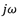for s.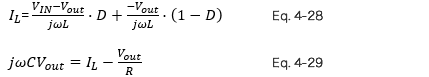Here, setting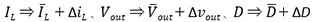and rearranging, we obtain the following.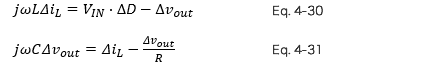These equations yield results similarly to the previously derived equations 4-26 and 4-27.

The basic conceptual procedure is:

① Consider the stable state of the system
1) The coil current does not change in one period
2) The capacitor charge amount does not change in one period
※At this time, express the impedance using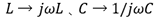② Set, and determineIn this way, if the basic principles are understood,can be determined comparatively simply.

This concludes our discussion of the state-space averaging method. Because the state-space averaging method is a method of approximation, it should always be kept in mind that the range of applicability and conditions for application of the method are important.